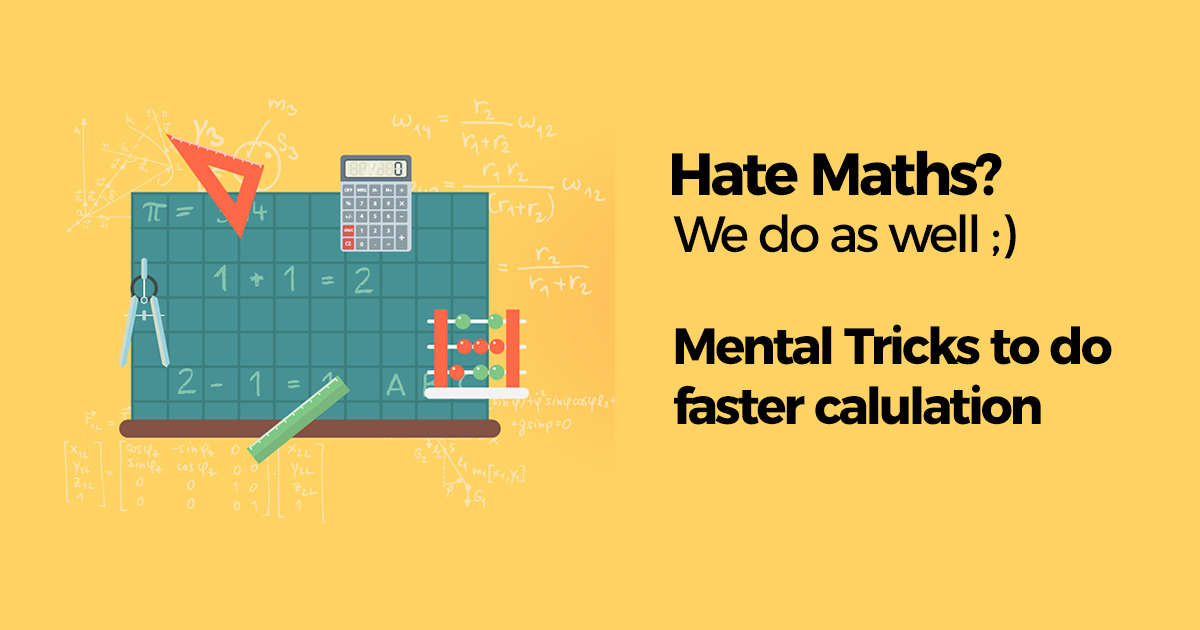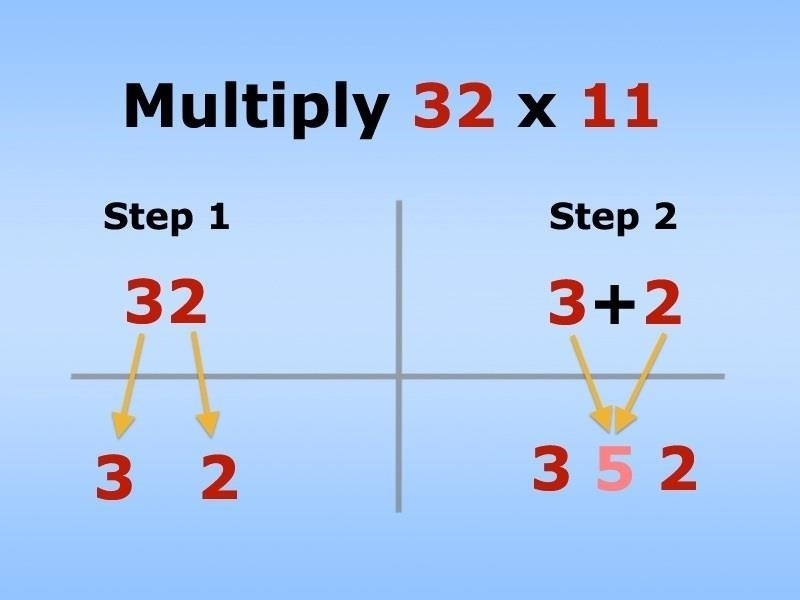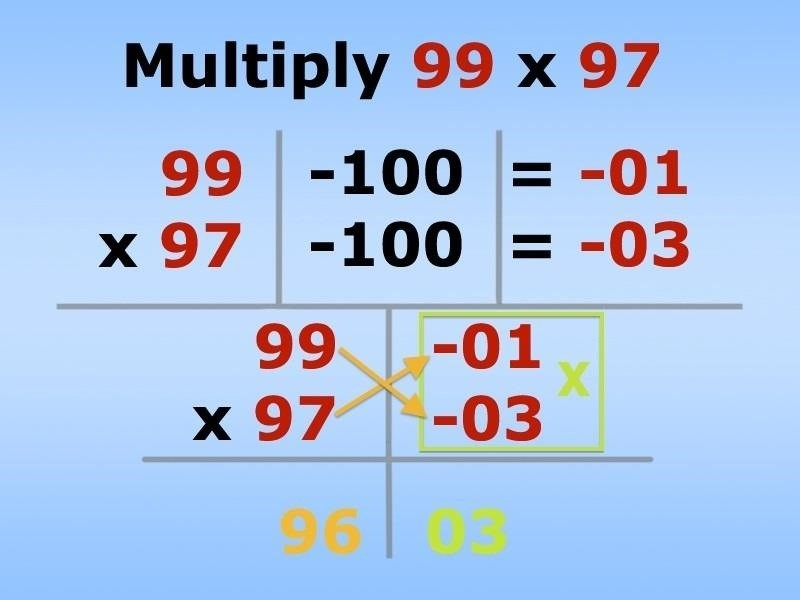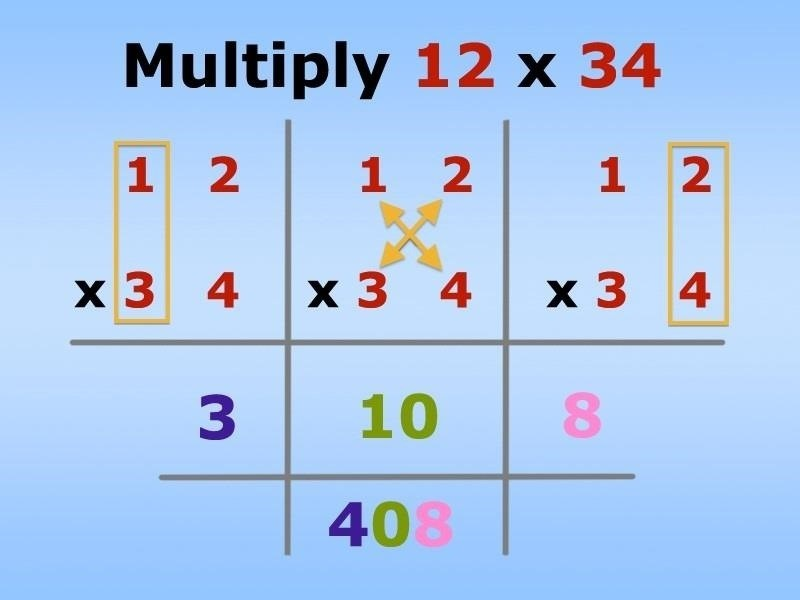###### 2+2=4

That’s about as much math as I can handle without a calculator on a daily basis. I literally hate doing math more than anything in life, mostly because I’m not good at it—and I hate doing things I’m not good at.

So, when I come across a cool math trick online that claims to make life easier for folks like me, I’m all ears. While calculus is (basically) useless for the average person’s everyday life, multiplication, addition, subtraction, and percentages are all things we should be able to do—and without a calculator.

### 1. How to Multiply Double-Digit Numbers by 11

My multiplication table stopped at 10, so beyond that, I’m making calculations based on my memory or counting in my head. However, using Vedic math, multiplying by 11 is a piece of cake.

All you need to do is add the digits of the number you are multiplying by 11 and place that in the middle of the original number. If the sum of the digits is 10 or larger, simply carry it over. Better to see it than me write it.See how easy that was? Basically, if you know how to add, you know how to multiply by 11.

Now, let’s look at another example.

• 11 x 11

Just separate the number being multiplied by 11 (in this case, also 11) so that there’s space for your number in-between. Now, just add the two digits in that number together (1 + 1 = 2) and put the sum in that space you left with. That gives you 121.

• 58 x 11

Just add 5 + 8, which gives you 13. The put it in-between the 5 and 8 and you get 5138. But, that’s not right, since you are left with a carry Go ahead and carry it over and you’ll end up with 638, that’s all.

### 2. How to Multiply Numbers Close to the Power of 10

This method is based on the uses of powers of 10 (tens, hundreds, thousands, etc.) and cross subtracts and multiplies the sums. Again, much better to see what I am saying then trying to read it. Here’s an example of multiplying two, two-digit numbers (a base of hundreds).• 99 x 97

Take the difference of each number from 100 and places those numbers in the right-hand column (99 – 100 = -01 and 97 – 100 = -03).

Then cross-adds one set of numbers (either pair would work) to get the first number of the answer. So, 99 + -03 or 97 + -01 = 96. So, that had been the first part of the answer.

Now multiply the two smaller numbers (-01 x -03) to get the second part of the answer. -01 x -03 = 03. So, that makes the answer 9603.

Pretty awesome right?

This same method works for any base often. 999 x 987 or 9,878 x 9,999 would all work using a base of 1,000 and 10,000 respectively.

### 3. How to Multiple Double-Digits by Any Other Double-Digits

The cool thing about math is watching how impossible combinations seem to come out perfectly in the end. By doing certain affairs, you can solve wildly complex equations into simple, step-by-step solutions.

Using the Vertical and Crosswise Pattern, we can easily multiply large two-digit numbers like the one pictured below.anding method of multiplication, we are going to separate and conquer.

• 12 x 34

First, we multiply vertically up the right side. 2 x 4 = 8. So, 8 will be the last digit in our answer.

Next, we cross-multiply. 3 x 2 = 6 and 4 x 1 = 4. Now add 6 + 4 to get 10. Carry over the 1 like you normally would, and you are left with 0, which will go in front of the 8 we already have.

So, as of now, you should have 08 in you answer line.

Lastly, we vertically multiply up the left side. 3 x 1 = 3 and add the carried 1. Place that in the front of our answer line and we get 408.

### 4. How to Multiply Using Lines Instead of Numbers

If you’re more observable learner that really hates numbers, you can also substitute digits in lines. I’m not going to explain this one—just watch and you’ll get it

###### Don’t You Love Math Now?

There are some really amazing math tricks using Vedic-style mathematics, so be sure to watch the full video to get a firmer grasp on it and start using it in your everyday life.